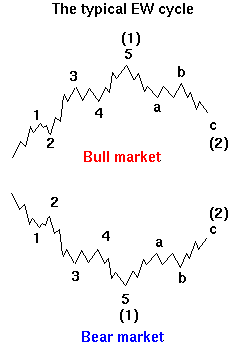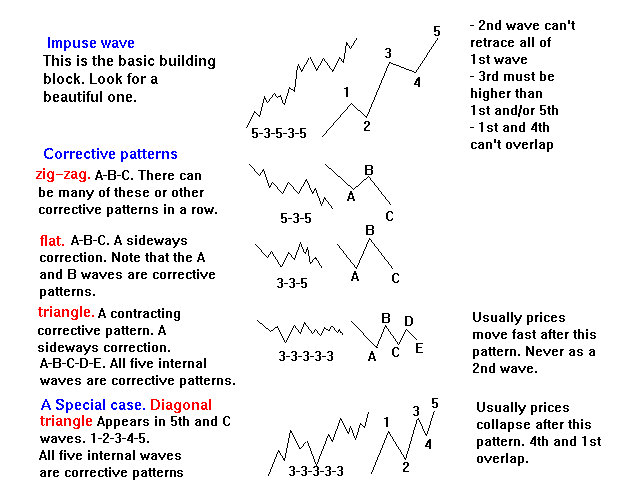### Elliott Wave TheoryThe Elliott Wave Theory was developed by Ralph Nelson Elliott in the 1930's. Elliott was born a U.S. citizen in 1871 and he died in 1948. The Elliott Waves describe the ebb and flow of collective mood. A bull market in collective mood is caused by our need to build a better future based on material values. A bear market is caused by our need to take a rest.

As long as there is fear that the bad old times will return, the bull market continues. Fearlessness is the condition for a cycle top.

As long as there is hope that the favorable trend will resume, the bear market continues. Hopelessness is the condition for a new cycle.

 EWT Basics A basic impulse wave consists of five internal waves (1-2-3-4-5) and a basic corrective pattern (zig-zag) consists of three internal waves (A-B-C). After an impulse wave comes a corrective pattern or a combination of them. If a correction consists of a series of corrective patterns then there must be an odd number of corrective patterns in a combination. Second and fourth corrective patterns are labeled 'X-waves'. After a correction comes an impulse wave or a diagonal triangle. A diagonal triangle can NEVER occur as the 3rd wave. Impulse Waves Wave 1 is an impulse wave. Wave 2 is a corrective pattern or a combination of corrective patterns. Wave 2 MUST retrace less than 100% of the 1st wave. Wave 3 is an impulse wave. Wave 3 MUST be higher than 1st and/or 5th wave. Wave 4 is a corrective pattern or a combiantion of corrective patterns. The end point of wave 4 MAY NOT overlap the 1st wave. Wave 5 is usually an impulse wave and sometimes a diagonal triangle. Corrective Waves There are three basic types of corrective patterns: a zig-zag, a flat and a triangle. Inside a corrective pattern in a zig-zag wave A is an impulse wave. wave B is a corrective pattern or a combination of corrective patterns. Wave B MUST retrace less than 100% of the A-wave. wave C is usually an impulse wave and sometimes a diagonal triangle. in a flat wave A is a zig-zag, flat or a combination of corrective patterns. wave B is a corrective pattern or a combination of corrective patterns. Wave B can retrace up to 162% of the A-wave. wave C is usually an impulse wave and sometimes a diagonal triangle. Wave C ends usually on the same level as A-wave. in a triangle waves A, B, C and D are a flat, zig-zag or a combination of corrective patterns. Wave E can be any corrective pattern or a combination of them. in a contracting triangle wave A is usually higher than wave B. wave B is ALWAYS higher than wave C. wave C is ALWAYS higher than wave D. wave D is ALWAYS higher than wave E. in an expanding triangle at least waves B, C and D are higher than the previous wave.Occurrence
• Impulse waves occur as
• 1st waves in impulse waves
• 3rd waves in impulse waves
• 5th waves in impulse waves
• A waves in zigzags
• C waves in zigzags
• C waves in flats
• Zigzags occur as
• 2nd waves in impulse waves
• 4th waves in impulse waves
• B waves in zigzags
• A waves in flats
• B waves in flats
• A waves in horizontal triangles
• B waves in horizontal triangles
• C waves in horizontal triangles
• D waves in horizontal triangles
• E waves in horizontal triangles
• 1st waves in diagonal triangles
• 2nd waves in diagonal triangles
• 3rd waves in diagonal triangles
• 4th waves in diagonal triangles
• 5th waves in diagonal triangles
• double zigzags, triple zigzags and other corrective combinations
• Flats occur as
• 2nd waves in impulse waves
• 4th waves in impulse waves
• B waves in zigzags
• A waves in flats
• B waves in flats
• A waves in horizontal triangles
• B waves in horizontal triangles
• C waves in horizontal triangles
• D waves in horizontal triangles
• E waves in horizontal triangles
• 1st waves in diagonal triangles
• 2nd waves in diagonal triangles
• 3rd waves in diagonal triangles
• 4th waves in diagonal triangles
• 5th waves in diagonal triangles
• Corrective combinations
• Horizontal Triangles occur as
• 4th waves in impulse waves
• B waves in zigzags
• B waves in flats
• E waves in horizontal triangles
• Corrective combinations
• Diagonal Triangles occur as
• 5th waves in impulse waves
• C waves in zigzags
• C waves in flats
Misc
• The same patterns occur both long-and short-term.
• Every wave in an EW pattern is an EW pattern or a combination of corrective EW patterns.
• The momentum peak is usually achieved in the 3rd and the C-wave.
• Third wave and C-wave is usually the highest wave in a pattern.
• A correction retraces most likely a golden ratio 38,2% or 61,8% of the previous impulse wave. The previous fourth wave of one lesser degree usually offers good support. Especially the end and the price extreme of the previous fourth wave are good bets for significant support.
• For most of the time there are several possible ways to count the waves. Sentiment and cycle analysis can help determining the correct count.
• A more complete but controversial list of EW rules and guidelines by Richard Swannell
• You can learn more about Elliott Wave Theory in A. J. Frost's and R. Prechter's classic Elliott Wave Principle

A clear EWT tutorial